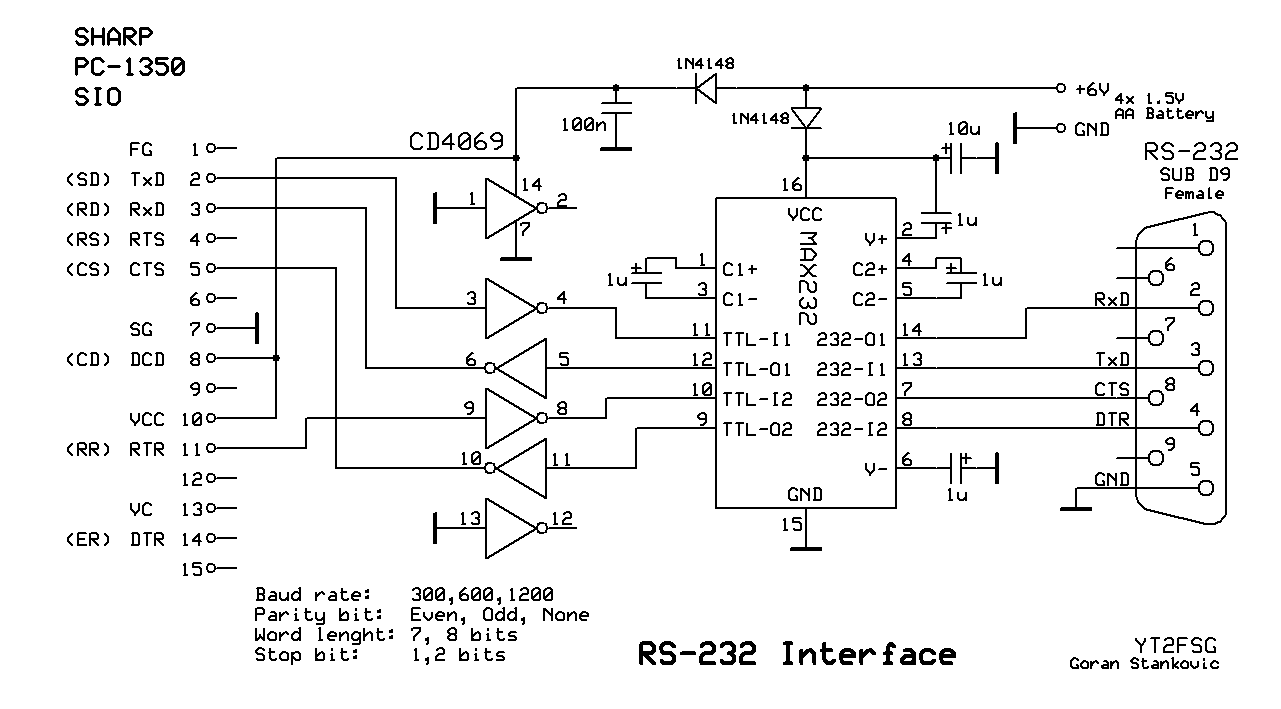# SHARP Pocket Computers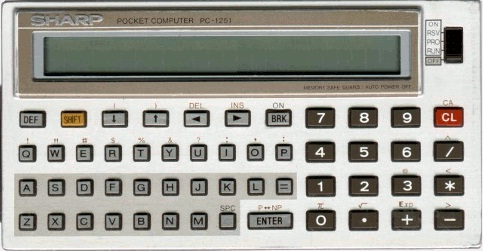Look of the display at the input matrix: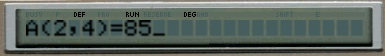## PC-1251

Start Mathematics Def:
M - Matrix multiplication
A - Matrix inversion
D - Determinant of the matrix
N - Numerical integration
F - Zero of a function
S - System of linear equations
X - System of nolinear equationsLook of the display at the graph of a function: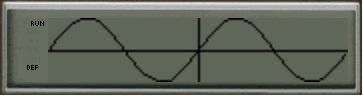## PC-1350

Start Mathematics Def:
M - Matrix multiplication
A - Matrix inversion
D - Determinant of the matrix
N - Numerical integration
J - Cubic equation
F - Zero of a function
G - Graph of a function
S - System of linear equations
X - System of nolinear equations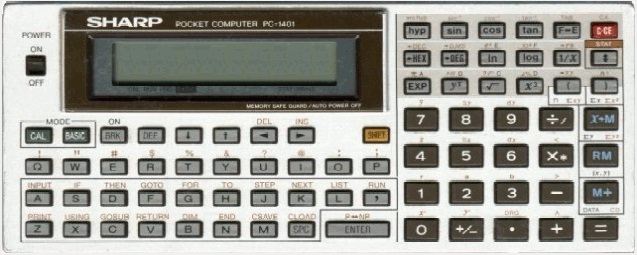Look of the display at the input matrix: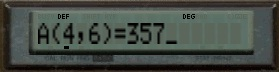## PC-1401

Start Mathematics Def:
M - Matrix multiplication
A - Matrix inversion
D - Determinant of the matrix
N - Numerical integration
F - Zero of a function
S - System of linear equations
X - System of nolinear equations## PC-1403

Start Mathematics Def:
N - Numerical integration
J - Cubic equation
F - Zero of a function

## BASIC Games for SHARP PC-1350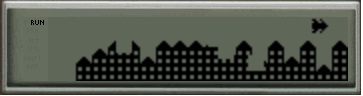Download Game: Bomber Start Game Def B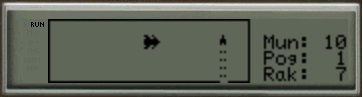Download Game: Rocket 1 Start Game Def Z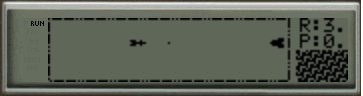Download Game: Rocket 2 Start Game Def X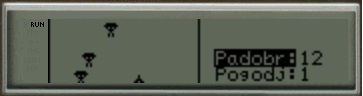Download Game: Paratroopers Start Game Def A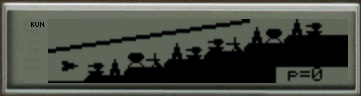Download Game: Scramble Start Game Def S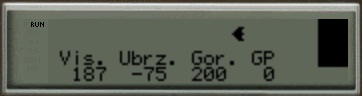Download Game: Landing on the Moon Start Game Def N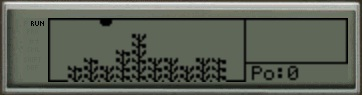Download Game: Weeds Start Game Def K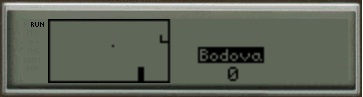Download Game: Basketball Start Game Def D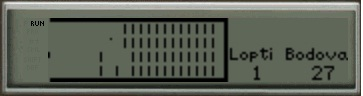Download Game: Ball Start Game Def F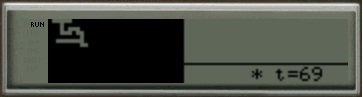Download Game: Labyrinth Init Game Def J Start Game Def L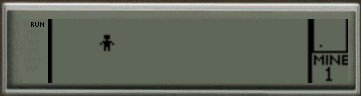Download Game: Mine Start Game Def MDownload Game: Cannon Start Game Def G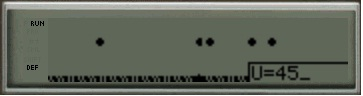Download Game: Meteors Start Game Def V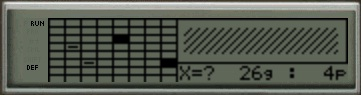Download Game: Submarines Start Game Def C Link YouTube Game Submarines

#### Link: AS61860 Assembler for SHARP Pocket Computers